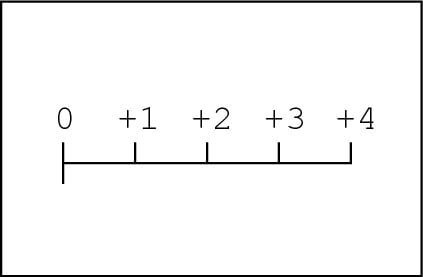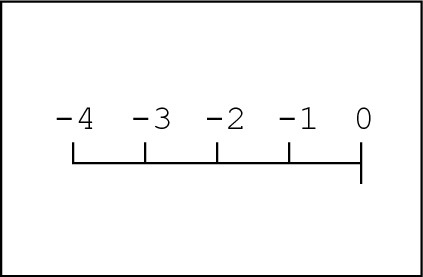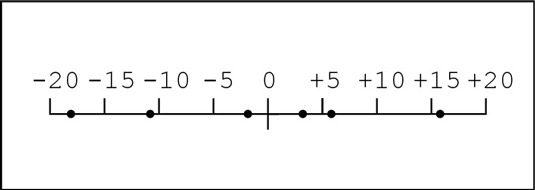##### Basic Math & Pre-Algebra For Dummies Book + Workbook BundlePositive and negative numbers are all integers. Integers are whole numbers that are either greater than zero (positive) or less than zero (negative). For every positive integer, there's a negative integer, called an additive inverse, that is an equal distance from zero.

You indicate a positive or negative number by using positive (+) and negative (–) signs. Although you don't have to include a positive sign when you write positive numbers, you must always include the negative sign when you write negative numbers.• Positive numbers are bigger, greater, or higher than zero. They are on the opposite side of zero from the negative numbers. Positive numbers get bigger and bigger the farther they are from zero: 81 is bigger than 25 because it’s farther away from zero; 212° F, the boiling temperature of water, is farther away from zero than 32° F, the temperature at which water freezes. They’re both positive numbers, but one is bigger than the other.• Negative numbers are smaller than zero. Negative numbers get smaller and smaller the farther they are from zero. This can get confusing because you may think that –400 is bigger than –12. But just think of –400° F and –12° F. Neither temperature is pleasant to think about, but –400° is definitely less pleasant — colder, lower, smaller. When dealing with negative numbers, the number closer to zero is the bigger number.

• Zero (0) has the unique distinction of being neither positive nor negative. Zero separates the positive numbers from the negative ones. In a line with zero in the middle, negative numbers line up on the left, and positive numbers line up on the right: –4, –3, –2, –1, 0 1, 2, 3, 4.

When you visualize negative and positive numbers in a line like this, it's easy to figure out which numbers are greater than others: For any two numbers in the line, the number to the right is always greater.• Additive inverses are numbers that are opposite in sign but the same otherwise. Two numbers are additive inverses of one another if their sum is zero: a + (–a) = 0. Additive inverses are always the same distance from zero (in opposite directions) on the number line. For example, the additive inverse of –6 is +6; the additive inverse of 1/5 is –1/5.